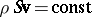# Continuity equation

One of the basic equations in hydrodynamics, expressing the law of conservation of mass for any volume of a moving fluid (or gas). In Euler variables the continuity equation has the form

$\frac{\partial \rho}{\partial t } + \text{div}(\rho \mathbf{v}) \equiv \frac{\partial \rho}{\partial t} + \frac{\partial (\rho v_x)}{\partial x} + \frac{\partial (\rho v_y)}{\partial y} + \frac{\partial (\rho v_z)}{\partial z} = 0,$

where $\rho$ is the density of the fluid, $\mathbf{v}$ is its velocity at a given point, and $v_x, v_y, v_z$ are the projections of the velocity on the coordinate axes. If the fluid is incompressible $(\rho = \text{const})$, then the continuity equation takes the form

$\text{div } \mathbf{v} = 0 \quad \text{or} \quad \frac{\partial v_x}{\partial x} + \frac{\partial v_y}{\partial y} + \frac{\partial v_z}{\partial z} = 0.$

For a stationary one-dimensional flow in a tube, canal, etc., with cross-sectional area $S$, the continuity equation gives the lawfor the flow.

How to Cite This Entry:
Continuity equation. Encyclopedia of Mathematics. URL: http://encyclopediaofmath.org/index.php?title=Continuity_equation&oldid=29562
This article was adapted from an original article by BSE-3 (originator), which appeared in Encyclopedia of Mathematics - ISBN 1402006098. See original article